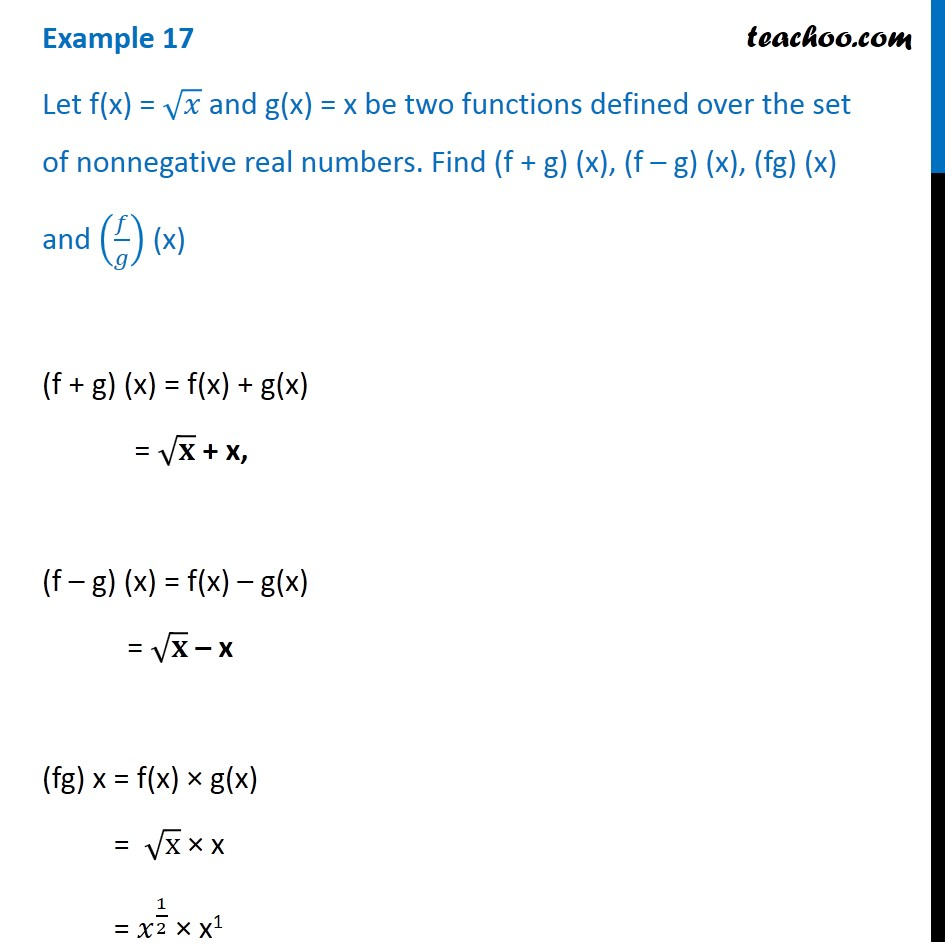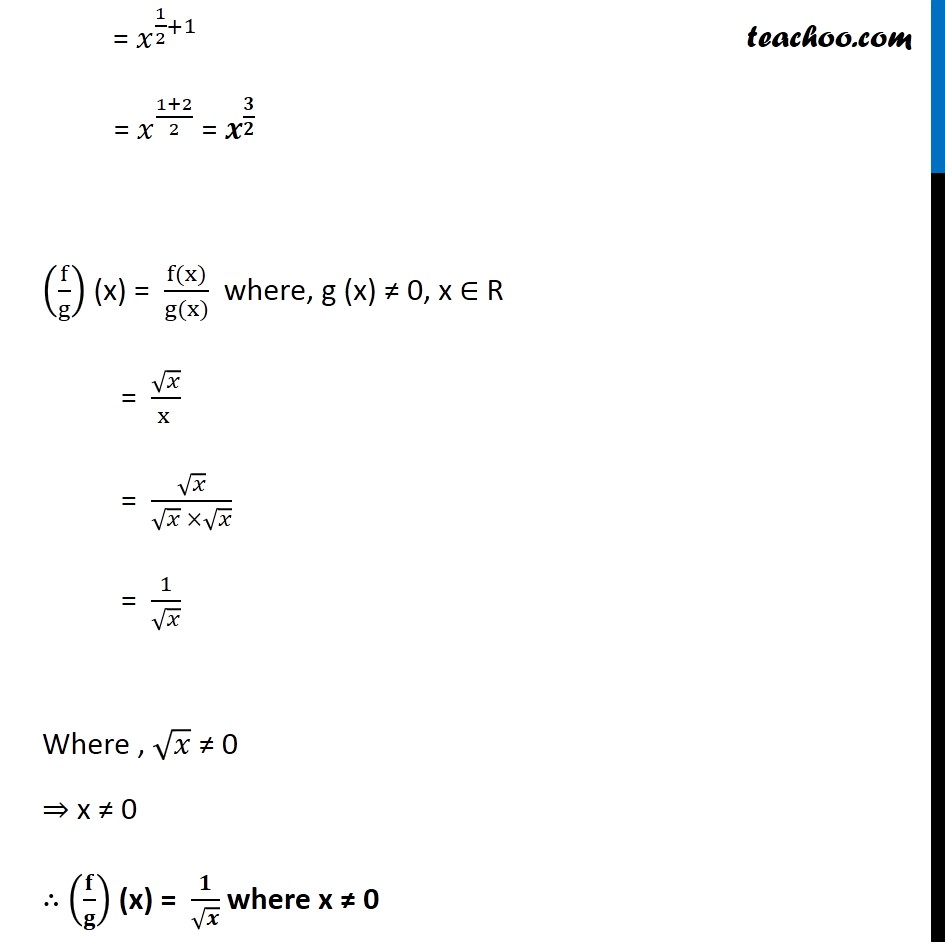Examples

Chapter 2 Class 11 Relations and Functions (Term 1)
Serial order wise### Transcript

Example 17 Let f(x) = √𝑥 and g(x) = x be two functions defined over the set of nonnegative real numbers. Find (f + g) (x), (f – g) (x), (fg) (x) and (𝑓/𝑔) (x) (f + g) (x) = f(x) + g(x) = √𝐱 + x, (f – g) (x) = f(x) – g(x) = √𝐱 – x (fg) x = f(x) × g(x) = √x × x = 𝑥^(1/2) × x1 = 𝑥^(1/2+1) = 𝑥^((1+2)/2) = 𝒙^(𝟑/𝟐) (f/g) (x) = (f(x))/(g(x)) where, g (x) ≠ 0, x ∈ R = √𝑥/(x ) = √𝑥/(√𝑥 ×√𝑥) = 1/√𝑥 Where , √𝑥 ≠ 0 ⇒ x ≠ 0 ∴ (𝐟/𝐠) (x) = 𝟏/√𝒙 where x ≠ 0